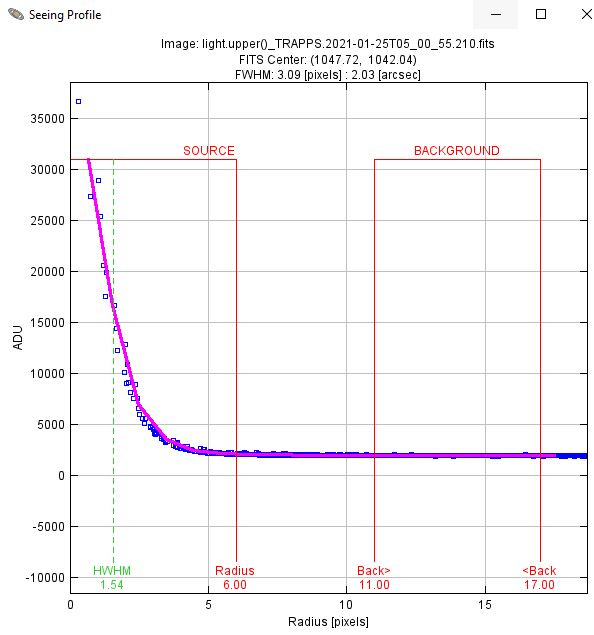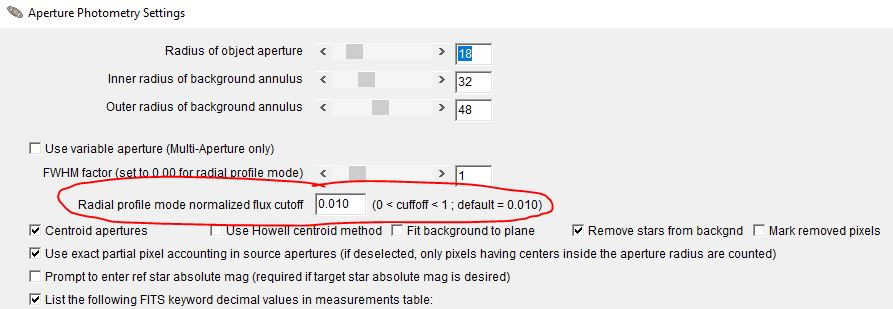# Auto Aperture Size Selection Algorithm in AIJ v5ClassicListThreaded1 messageAdministrator This post was updated on . AIJ v5 uses the "Seeing Profile" (or Radial Profile) function from AIJ v3/4 to (optionally) automatically extract aperture sizes. The AIJ paper description of how the aperture radii are selected is now outdated. The paper describes the radius extraction in Appendix A.4 as: "The aperture radius is set to 1.7 × FWHM, the inner radius of the sky-background annulus is set to 1.9 × FWHM, and the outer radius of the sky-background annulus is set to 2.55 × FWHM. These radii give an equal number of pixels in the aperture and sky-background annulus." The revised approach is as follows: A "Radial profile mode normalized flux cutoff" (let's call it Fcut) is defined. The auto aperture radius that is extracted is the radius at which the flux in the radial profile drops below Fcut relative to the normalized central bin of the radial profile. The default value of Fcut is 0.01, so the default automatically selected aperture radius is the radius at which the flux in the radial profile drops by 99% compared to the central measurement of the radial profile. Another way of saying it is that the aperture size selected is the distance from the center of the aperture where the azimuthally (circularly) averaged flux has dropped by 99% compared to the central bin of the radial profile. Note that the flux measurements are compared to the central bin of azimuthally averaged flux, which is related to the peak value, but is not necessarily the peak value since more than one pixel could be inside the central bin. Find an example from a sharp-focused image below. Note that the FWHM is 3.09, and the radius selected is 6 pixels (which turns out to be 1.94 x the FWHM). The point is that the selected radius is the distance from the center of aperture where the average flux at that distance (i.e. averaged all the way around the star) drops to 99% of the highest bin. Once r1 is found as described above, the background annulus radii r2 and r3 are defined using simple multipliers: r1 = aperture radius extracted as described above r2 = r1 * 1.75 r3 = r2 * 1.5 (the aperture radii are rounded UP to the next highest integer - i.e. the ceiling function) Keep in mind that in all cases, the measurement is extracted from the image where you click to place the initial aperture. For time-series photometry, a larger or smaller aperture may be needed if seeing and/or focus are highly variable compared to the initial image.Although the default Fcut is 0.01, it can be changed in the Aperture Settings panel in the "Radial profile mode normalized flux cutoff" box. A smaller value (such as 0.005) will produce a larger automatically extracted aperture. A larger value (e.g 0.02) would result in a smaller automatically selected aperture size.